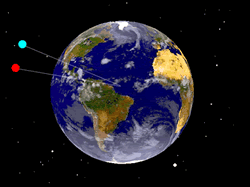Here is the way that Einstein's Equivalence Principle is often introduced:

We are in a room without windows, and so can not see out. No experiment that measures only properties inside the room can determined whether the room is:

• On the surface of the earth where the acceleration due to gravity is down and equal to 9.80 m/s2.
• On a rocket ship in free space that is accelerating upwards at 9.80 m/s2.

Since there is no measurable difference between these two circumstances, there is no difference. Thus acceleration is equivalent to a gravitational field.

This small document will refine this argument.

 One possible experiment is to drop two balls. In both cases they will accelerate down at 9.80 m/s2.However, when objects fall due to the gravity of the earth, they fall towards the centre of the earth, as shown. Thus, if we watch two objects falling under the influence of gravity we will see them moving towards each other.Close to the surface of the earth, it is difficult to measure that the two objects are approaching each other and not just falling straight down. However, that is what they are really doing. Thus the first figure above is what would happen if the room were on the rocket ship accelerating upwards. If the room were on the Earth the two acceleration vectors shown will be pointing very very slightly towards each other.

This does not mean that the Equivalence Principle is wrong; it means that the principle is only true for points in spacetime, not regions. In physics-speak, the Equivalence Principle is local.

Since the General Theory of Relativity is based on the Equivalence Principle, this means that the theory is also local: it describes the trajectories, the "worldlines," of objects only in terms of the local geometry of spacetime.

So we must slightly modify our original specification of the experiment:

We are in a room without windows, and so can not see out. No experiment that measures only local properties inside the room can determined whether the room is on the surface of earth or in free space and accelerating upwards.

There is one other small thing that we have ignored: the fact that the two masses attract each other gravitationally and will thus move towards each other even on the rocket ship. But on the Earth they will move towards each other at a faster rate than on the rocket ship.